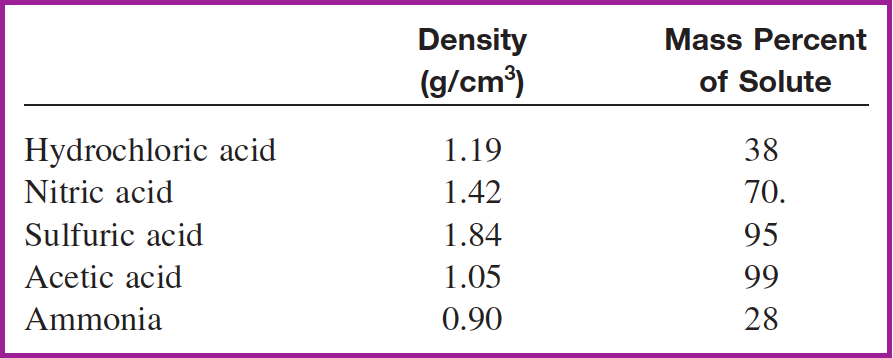# Problem: Common commercial acids and bases are aqueous solutions with the following properties:Calculate the molarity, molality, and mole fraction of each of the preceding reagents.

###### FREE Expert Solution
98% (347 ratings)
###### Problem Details

Common commercial acids and bases are aqueous solutions with the following properties:Calculate the molarity, molality, and mole fraction of each of the preceding reagents.

Frequently Asked Questions

What scientific concept do you need to know in order to solve this problem?

Our tutors have indicated that to solve this problem you will need to apply the Mole Fraction concept. You can view video lessons to learn Mole Fraction. Or if you need more Mole Fraction practice, you can also practice Mole Fraction practice problems.

What textbook is this problem found in?

Our data indicates that this problem or a close variation was asked in Chemistry: An Atoms First Approach - Zumdahl Atoms 1st 2nd Edition. You can also practice Chemistry: An Atoms First Approach - Zumdahl Atoms 1st 2nd Edition practice problems.